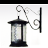# Raster To Polygon Failure

2304
1
03-09-2014 08:51 PMNew Contributor III
Hello all, i'm running into a problem i haven't been able to solve. I'm running ArcGIS 10.0

Here is a sample of the code i'm currently running.

`import arcpy from arcpy import env  #Establish Workspace env.workspace = "C:/Fakepath.gdb"  #Set Variables DATE = arcpy.GetParameterAsText(0) # DDMMMYY, MMM = 3 day abbreviation for month, March = Mar  #Integer Conversion INTHEAT = arcpy.gp.Int_sa("MULT" + DATE, "INT" + DATE") arcpy.AddMessage("We now have only whole numbers")  arcpy.RasterToPolygon_conversion("INT" + DATE, r'Processed_Points\POLY' + DATE, "SIMPLIFY", "") arcpy.AddMessage("Converted Raster to Polygon") `

The script is much longer and complex however it has been failing at Raster To Polygon, displaying this error.

`<class 'arcgisscripting.ExecuteError'>:ERROR 010151: No features found in C:\Fakepath.gdb\Processed_Points\POLY10MAR14. Possible empty feature class.`

Now i've had this problem before in model builder where this tool was originally built. I decided to switch to python as I prefer it over model builder. The model failed in the exact same spot. Now when i run the tool from the arctoolbox the tool runs fine. I should also mention that RasterToPolygon does generate the polygon. That polygon and the one that is generated separately via arctoolbox are the exact same. Anyone have any ideas what is going on here?
Tags (3)
1 Solution

Accepted SolutionsNew Contributor III
UPDATE:

So I tried something different that worked. It seems that I cannot make the destination of the polygon into the same location as the raster. In order to circumvent this I created a scratch geodatabase where the polygon is saved. The script ran fine, i'm going to add the copy feature tool and see if that works. It is an odd work around. Has anyone else experienced this problem?

UPDATE 2:
So I figured out a work around. Here is the code I ended up using.

`import arcpy from arcpy import env  #Establish Workspace env.workspace = "C:/Fakepath.gdb"  #Set Variables DATE = arcpy.GetParameterAsText(0) # DDMMMYY, MMM = 3 day abbreviation for month, March = Mar  #Integer Conversion INTHEAT = arcpy.gp.Int_sa("MULT" + DATE, "INT" + DATE") arcpy.AddMessage("We now have only whole numbers")  #Raster to Polygon arcpy.RasterToPolygon_conversion(INTHEAT, r'C:\Fakepath\scratch.gdb\HPOLY' + DATE, "SIMPLIFY", "") arcpy.CopyFeature(r'C:\Fakepath\scratch.gdb\HPOLY' + DATE, r'Processed_Data\HPOLY' + DATE) arcpy.Delete(r'C:\Fakepath\scratch.gdb\HPOLY') arcpy.AddMessage("Converted Raster to Polygon")``import arcpy from arcpy import env  #Establish Workspace env.workspace = "C:/Fakepath.gdb"  #Set Variables DATE = arcpy.GetParameterAsText(0) # DDMMMYY, MMM = 3 day abbreviation for month, March = Mar  #Integer Conversion INTHEAT = arcpy.gp.Int_sa("MULT" + DATE, "INT" + DATE") arcpy.AddMessage("We now have only whole numbers")  #Raster to Polygon arcpy.RasterToPolygon_conversion(INTHEAT, r'C:\Fakepath\scratch.gdb\HPOLY' + DATE, "SIMPLIFY", "") arcpy.CopyFeature(r'C:\Fakepath\scratch.gdb\HPOLY' + DATE, r'Processed_Data\HPOLY' + DATE) arcpy.Delete(r'C:\Fakepath\scratch.gdb\HPOLY') arcpy.AddMessage("Converted Raster to Polygon")`